# NUST Mathematics MCQs Chapter 6

NUST entry test 2023 NET contains most of the Mathematics MCQs. FSC Part 1 Math book MCQs. HSSC Mathematics questions MCQs. Chapterwise mathematics MCQs for first year 11 class MCQs.

188
Created on By Ali Durrani

Math Ch 6 Sequence and Series

1 / 30

If sum of series is not defined then it is called:

2 / 30

If sum of series is defined then it is called:

3 / 30

. An infinite geometric series is divergent if

4 / 30

An infinite geometric series is convergent if

5 / 30

The sum of infinite geometric series is valid if

6 / 30

The sum of terms of a sequence is called:

7 / 30

An arrangement of numbers according to some definite rule is called:

8 / 30

A sequence is also known as:

9 / 30

A sequence is function whose domain is

10 / 30

The last term of an infinite sequence is called :

11 / 3012 / 30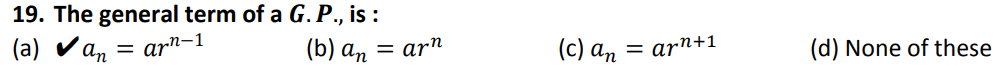13 / 30

No term of a 𝑮. 𝑷., is:

14 / 3015 / 3016 / 30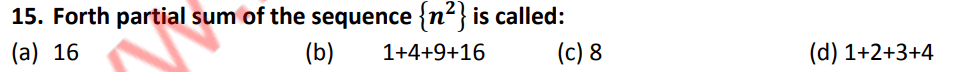17 / 30

The sum of terms of a sequence is called:

18 / 30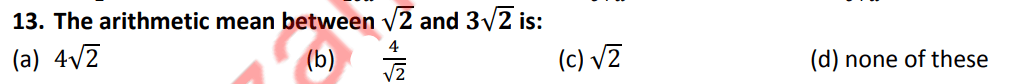19 / 3020 / 3021 / 3022 / 30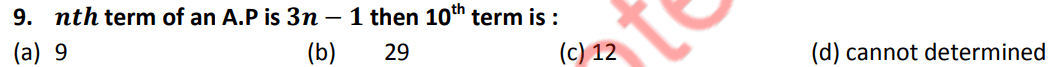23 / 30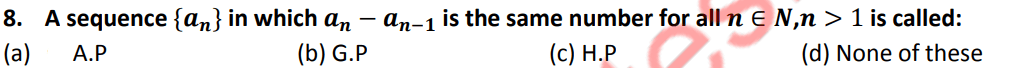24 / 30

The next term of the sequence 𝟏, 𝟐, 𝟏𝟐, 𝟒𝟎, … is

25 / 3026 / 30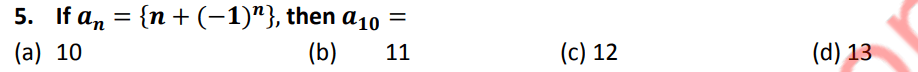27 / 30

4. As sequence whose range is 𝑹 𝒊. 𝒆., set of real numbers is called:

28 / 30

3. A sequence is function whose domain is

29 / 30

2. A sequence is also known as:

30 / 30

An arrangement of numbers according to some definite rule is called:

The average score is 57%

0%

### 2 responses to “NUST Mathematics MCQs Chapter 6”

1. Shahzaib khan says:

Why the question are not complete olz answer

Salam